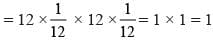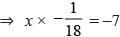## 20 Questions MCQ Test Mathematics Olympiad Class 7 | Olympiad Test: Integers -1

Description
Attempt Olympiad Test: Integers -1 | 20 questions in 40 minutes | Mock test for Class 7 preparation | Free important questions MCQ to study Mathematics Olympiad Class 7 for Class 7 Exam | Download free PDF with solutions
QUESTION: 1

### The sum of two integers is – 17. If one of them is 15, then what is the other?

Solution:

15 + x = –17 ⇒ x = –17 –15 = –32.

QUESTION: 2

### The difference of an integer P and (–8) is 6. What is the value of P?

Solution:

P – (–8) = 6
⇒ P + 8 = 6 ⇒ P = 6 – 8 = –2

QUESTION: 3

### What is the additive inverse of [ –5+7–2–3]?

Solution:

–5 + 7 –2 –3 = –10 + 7 = –3
Additive inverse of –3 = 3

QUESTION: 4

If the sum of –1037 and 693 is subtracted from –45, then which value will be obtained?

Solution:

(–45)–(–1037 +693)
= –45 – (–344) = –45 + 344 = 299

QUESTION: 5

The sum of two integers is 67. If one of them is –43, then what is the other?

Solution:

(–43) + x = 67 ⇒ x = 67 +43 = 110

QUESTION: 6

Find the value of (–2)×(–3)×(4)(–7).

Solution:

(–2) (–3) (4) (–7) = (24)(–7) = – 168

QUESTION: 7

What is the value of 8×(–7) + (–7)×9?

Solution:

8(–7) + (–7)9 = –56 –63 = –119

QUESTION: 8

What should be subtracted from –2 to get –17?

Solution:

(–2) – x = –17.⇒ x = –2 + 17 = 15

QUESTION: 9

Find the product of sum of –6 and 17 and difference of –12 and 15.

Solution:

Product = (–6 + 17) × [ 15 – (–12)]
= (11) × (+27) = 297

QUESTION: 10

What is additive inverse of [(–2)×(–7)]?

Solution:

(–2) × (–7) = 14

QUESTION: 11

By how much 5 exceed –5?

Solution:

–5 + x = 5 ⇒ x = 5 + 5 = 10

QUESTION: 12

How much less than –2 is –6?

Solution:

–2 – x = –6
⇒ x = –2 + 6 = 4

QUESTION: 13

What is the value of (–3) ×(–7) –12 + 15?

Solution:

(–3) × (–7) – 12 + 15
= 21 –12 + 15 = 36 – 12 = 24

QUESTION: 14

What is the value of 16 ÷(–2)×(– 4) –3 + 8?

Solution:

16 ÷(–2) × (–4) – 3 + 8
= (–8) × (–4)–3 + 8
= 32 – 3 + 8 = 40 – 3 = 37

QUESTION: 15

12÷12×12÷12=?

Solution:

12 ÷ 12 × 12 ÷12QUESTION: 16

12×12–12+12÷12–12=?

Solution:

12 × 12 – 12 + 12 ÷12 – 12
= 12 × 12 – 12 + 1 – 12
= 144 – 12 + 1 – 12
= 145 – 24 = 121

QUESTION: 17

27÷27–27+27–27×27=?

Solution:

27 ÷ 27 – 27 + 27 – 27 × 27
= 1– 27 + 27 – 729
= 28 – 756 = –728

QUESTION: 18

(–25)×8+(–25)×(12)=?

Solution:

(–25) × 8 + (–25) × 12
= (–25) [8 + 12]
= –25 × 20 = –500

QUESTION: 19

(17)×(–12)+(19)×(–12)

Solution:

17 × (–12) + (19) (–12)
= (17 + 19) (–12) = (36)(–12) = –432

QUESTION: 20

(?)÷(–18) = –7

Solution:

x ÷ (–18) = –7⇒ x = (– 7) × (–18) = 126Use Code STAYHOME200 and get INR 200 additional OFF Use Coupon Code